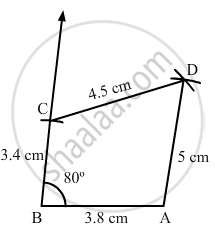Share

# Construct a Quadrilateral Abcd in Which Ab = 3.8 Cm, Bc = 3.4 Cm, Cd = 4.5 Cm, Ad = 5 Cm and ∠B = 80°. - Mathematics

Course
ConceptConstructing a Quadrilateral When Two Adjacent Sides and Three Angles Are Known

#### Question

Construct a quadrilateral ABCD in which AB = 3.8 cm, BC = 3.4 cm, CD = 4.5 cm, AD = 5 cm and ∠B= 80°.

#### Solution

Steps of construction:

Step I: Draw AB = 3 . 8 cm .

$\text { Step II: Construct} \angle ABC = 80^\circ .$

Step III : With B as the centre and radius 3 . 4 cm, cut off BC = 3 . 4 cm .

Step IV: With C as the centre and radius 4 . 5 cm, draw an arc .

Step V : With A as the centre and radius 5 . 3 cm, draw an arc to intersect the arc drawn in Step IV at D .

Step VI: Join AD, BC and CD to obtained the required quadrilateral .Is there an error in this question or solution?

#### APPEARS IN

RD Sharma Solution for Mathematics for Class 8 by R D Sharma (2019-2020 Session) (2017 to Current)
Chapter 18: Practical Geometry (Constructions)
Ex. 18.3 | Q: 1 | Page no. 8
Solution Construct a Quadrilateral Abcd in Which Ab = 3.8 Cm, Bc = 3.4 Cm, Cd = 4.5 Cm, Ad = 5 Cm and ∠B = 80°. Concept: Constructing a Quadrilateral - When Two Adjacent Sides and Three Angles Are Known.
S